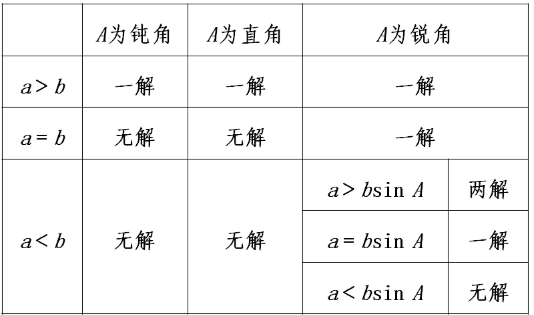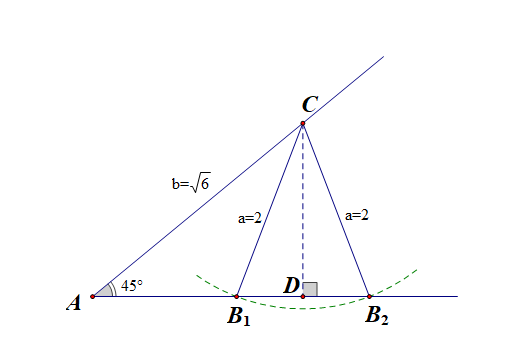# 判断三角形个数

## 前言

①代数法：从数的角度思考，根据大边对大角的性质，三角形内角和公式，正弦函数值判断；

②几何图形法，从形的角度思考，根据条件画出图形，通过图形直观判断三角形的个数；

## 情形列举

$$\triangle ABC$$中，已知$$a，b，A$$，三角形的解的个数比较复杂，见下表## 典例剖析

$$\triangle ABC$$中，已知$$a=2$$$$b=\sqrt{6}$$$$\angle A=45^{\circ}$$，则满足条件的三角形有【】个。

$A.1$ $B.2$ $C.0$ $D.无法确定$

$$B=60^{\circ}$$$$B=120^{\circ}$$，则对应的三角形有两个，故选$$B$$$$\Delta ABC$$中，已知$$b=40$$$$c=20$$$$\angle C=60^{\circ}$$，则此三角形$$\Delta ABC$$的解的情况是如何的？$$\cfrac{b}{sinB}=\cfrac{c}{sinC}$$，得到$$sinB=\cfrac{bsinC}{c}=\sqrt{3}>1$$$$k\in(0，6\sqrt{3})$$时，满足题意的三角形不存在；

$$k=6\sqrt{3}$$时，满足题意的三角形是唯一的，且是直角三角形。

$$k\in(6\sqrt{3}，12)$$时，满足题意的三角形是两个。

$$k=12$$时，满足题意的三角形是一个，是等腰三角形。

$$k>12$$时，满足题意的三角形是一个。

【解后反思】1、学生对这类题目的掌握一般都不太好，不会作图，不会应用图像解决问题。

2、这类题目作图的顺序是这样的，先做出$$\angle B$$，一条已知边$$BC$$要么水平放置，要么斜放着，一般都是斜放着，此时点$$C$$就有了着落，这样放置也便于求点$$C$$到下底边上的高，然后以点$$C$$为圆心，以$$AC$$长为半径画弧，若所画的弧与下底边有交点，这个交点就是点$$A$$，有几个交点就意味着有几个三角形存在，若所画的弧与下底边没有交点，则这样的三角形是不存在的。

$$k\times\cfrac{\sqrt{3}}{2}>12$$时，解得$$k>8\sqrt{3}$$，此时三角形是不存在的；

$$12$$等于点$$C$$到边$$AB$$的高度$$k\times\cfrac{\sqrt{3}}{2}$$时，

$$12=k\cfrac{\sqrt{3}}{2}$$，解得$$k=8\sqrt{3}$$，三角形是唯一的；$$12$$大于点$$C$$到边$$AB$$的高度$$k\cdot\cfrac{\sqrt{3}}{2}$$时，三角形是两个的，

$$12>k\times \cfrac{\sqrt{3}}{2}$$，解得$$k<8\sqrt{3}$$

$$12$$大于或等于边$$BC$$时，三角形是唯一的，即$$0<k\leqslant 12$$

【解后反思】①动静互换，体现了思维的灵活性；②是否可以这样想，有一种从形入手分析的思路，必然就会有一种从数入手的思路与之对应。

posted @ 2019-12-18 10:28  静雅斋数学  阅读(1827)  评论(1编辑  收藏  举报

----静雅斋